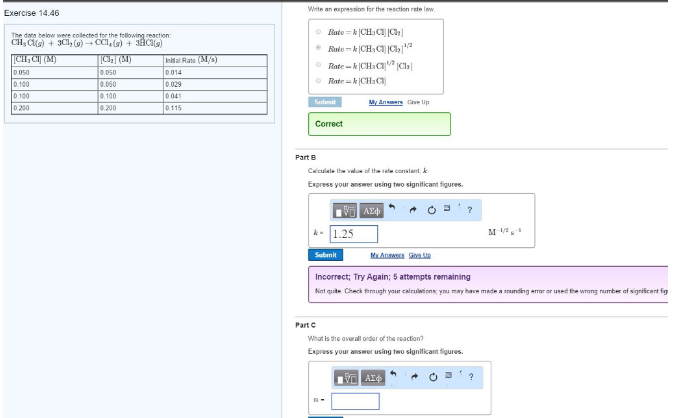# Problem: The data below were collected for the following reaction: CH3Cl(g) + 3Cl2 (g) → CCl4 (g) + 3HCl (g) Part A Write an expression for the reaction rate law. Part BCalculate the value of the rate constant, k. Express your answer using two significant figures. Part CWhat is the overall order of the reaction? Express your answer using two significant figures.

###### FREE Expert Solution
99% (75 ratings)###### Problem Details

The data below were collected for the following reaction:

CH3Cl(g) + 3Cl2 (g) → CCl4 (g) + 3HCl (g)

Part A

Write an expression for the reaction rate law.

Part B

Calculate the value of the rate constant, k. Express your answer using two significant figures.

Part C

What is the overall order of the reaction? Express your answer using two significant figures.